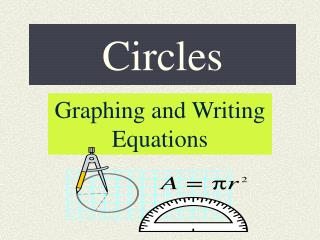DownloadDownload PresentationCircles

# Circles

Download Presentation## Circles

- - - - - - - - - - - - - - - - - - - - - - - - - - - E N D - - - - - - - - - - - - - - - - - - - - - - - - - - -
##### Presentation Transcript

1. Circles Graphing and Writing Equations

2. What is a circle? • A conic formed when…. • A second degree equation… • A locus of points…

3. Definition as a conic A circle is a conic or a conic section because it is formed by the intersection of a plane and a double-napped cone.

4. Algebraic Definition A circle is defined as the second degree equation Ax2+Bxy+Cy2 +Dx+Ey+F = 0 when A = C.

5. A circle is a locus of points….. But, what is a locus…? A locusis the set of all points, and only those points, that satisfy one or more conditions. So, “ A locus of points in a plane 10 cm from a point A” gives a geometric description of a circle with center A and a radius of 10

6. Geometric Definition A circle is thelocus or collection of all points (x, y) that are equidistant from a fixed point, (h, k) called the center of the circle. The distance r , between the center and any point on the circle is the radius

7. Using the distance formula, you can derive the standard form of a circle. (x- h)2 + (y- k)2 = r 2 where, center = (h, k) and radius = r

8. Equation of a circle: Practice Problems to write the equation of a circle when given the center and the radius.

9. What if the center and radius are not given? • Try these : • Center = (0,3) and solution point = (0, 6) • Endpoints of diameter are (-2,3) and (6,5) • Center on the line y = 2, and tangent to the x-axis at (3,0)

10. Graph the circle To graph a circle, locate all the points that are a fixed distance r, from the center (h, k). Click here to graph a circle

11. Graph a circle with center at the origin Example: Graph x2 + y2 = 4

12. To Graph a Circlecenter (h,k) and radius r Example: (x-3)2+   (y-3)2 =4 Click HereTo graph a circle if given a radius and a center

13. What are some applications? • Circular orbits of the earth for satellites. • Perfect shape for cross-section of a submarine. • Gears • Other